### Reasoning - Picture Questions

##### Directions: In the questions given below, four alternatives are given for each question. Click on the option which you think is the RIGHT ANSWER to the question.

Which number will come in the place of question mark (?)

1.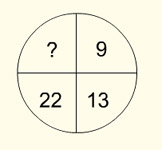Explanation: 22 is the sum of 13 and 9, similarly 44 is the sum of 22, 13 and 9.

2.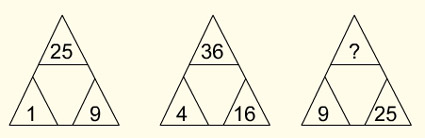Explanation: The first triangle contains squares of 1, 3 and 5, the second triangle contains squares of 2, 4 and 6 and the third triangle contains squares of 3, 5 and 7.

3.Explanation: The 4 quadrants contain cubes of 1, 2, 3 and 4.

4.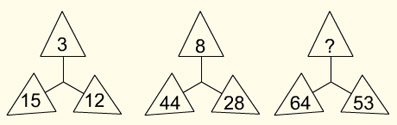Explanation: The number in the top triangle is 1/9 th of the sum of the two numbers at the bottom. In the first figure, 15 + 12 = 27/9 = 3, in the second figure 44 + 28 = 72/9 = 8 and thus in the question figure, 64 + 53 = 117/9 = 13.

5.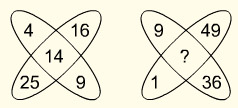Explanation: The number in the centre is the sum of square roots of the numbers surrounding it.

6.Explanation: The number in the centre is the sum of square roots of the numbers surrounding it divided by 5. In the first figure, square roots of the numbers are 5 + 5 + 10 + 10 = 30 ÷ 5 = 6. Thus in the question figure, the square roots of the number are 7 + 3 + 4 + 6 = 20 ÷ 5 = 4.

7.Explanation: The number in the centre is the product of the difference between the opposite numbers. In the first figure, 22 – 16 = 6 and 15 – 9 = 6. The number in the centre is 6 x 6 = 36.

8.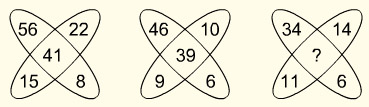Explanation: The number in the centre is the difference between the difference of opposite numbers. In the first figure, 56 – 8 = 48 and 22 – 15 = 7. The number in the centre is 48 – 7 = 41.

9.Explanation: The number in the centre is the sum of the product of the opposite numbers. In the first figure, 3 x 3 = 9 and 5 x 6 = 30. The number in the centre is 30 + 9 = 39.

10.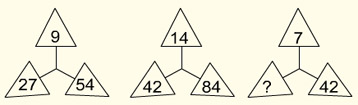Explanation: In the first figure, 9 x 3 = 27 and 9 x 6 = 54. In the second figure, 14 x 3 = 42 and 14 x 6 = 84. Similarly in the third figure, 7 x 3 = 21 and 7 x 6 = 42.

11.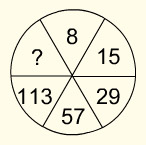Explanation: The numbers are in the sequence 8 + 7 = 15, 15 + 14 = 29, 29 + 28 = 57, 57 + 56 = 113 and 113 + 112 = 225.

12.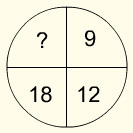Explanation: The numbers are in the sequence 9 + 3 = 12, 12 + 6 = 18 and 18 + 9 = 27.

13.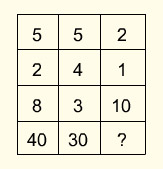Explanation: The number in the last row is half the product of numbers in each row. Thus in the first column 5 x 2 x 8 = 80 ÷ 2 = 40.

14.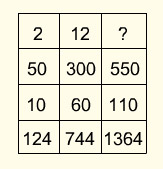Explanation: The number in the last row is double the sum of numbers in each row. Thus in the first column 2 + 50 + 10 = 62 x 2 = 124.

15.Explanation: The numbers are squares of 2, 4, 8 and 16.